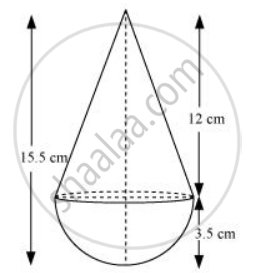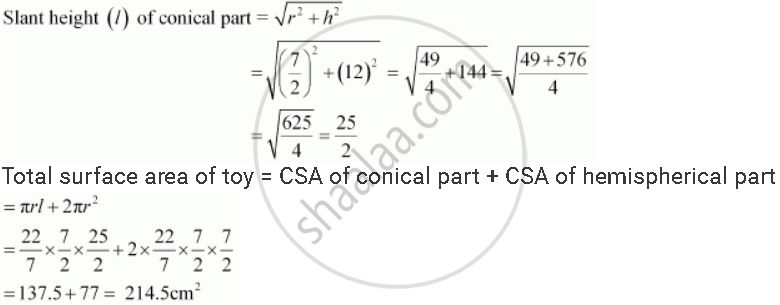Share
Notifications

View all notifications
Books Shortlist
Your shortlist is empty

# Solution for A Toy is in the Form of a Cone of Radius 3.5 Cm Mounted on a Hemisphere of Same Radius. the Total Height of the Toy is 15.5 Cm. Find the Total Surface Area of the to - CBSE Class 10 - Mathematics

Login
Create free account

Forgot password?
ConceptSurface Area of a Combination of Solids

#### Questions

A toy is in the form of a cone of radius 3.5 cm mounted on a hemisphere of same radius. The total height of the toy is 15.5 cm. Find the total surface area of the toy [Use π =22/7]

A toy is in the form of a cone of radius 3.5 cm mounted on a hemisphere of same radius on its circular face. The total height of the toy is 15.5 cm. Find the total surface area of the toy

#### Solution 1It can be observed that the radius of the conical part and the hemispherical part is same (i.e., 3.5 cm).

Height of hemispherical part = Radius (r) = 3.5 = 7/2 cm

Height of conical part (h) = 15.5 − 3.5 = 12 cm#### Solution 2

We have to find the total surface area of a toy which is a cone surmounted on a hemisphere.
Radius of hemisphere and the base of the cone (r) = 3.5cm

Height of the cone,

h = 15.5 - 3.5 = 12 cm

slant height (l) = sqrt(h^2 + r^2)

= sqrt(12^2 + 3.5^2)

= sqrt(156.25)

= 12.5 cm

So total surface are of toy

S = πrl + 2πr2

= πr(l + 2r)

= 22/7 xx 3.5 (12.5 +  2 xx 3.5)

= 214.5 cm2

Is there an error in this question or solution?

#### APPEARS IN

RD Sharma Solution for 10 Mathematics (2018 to Current)
Chapter 14: Surface Areas and Volumes
Q: 18
NCERT Solution for Mathematics Textbook for Class 10 (2014 to Current)
Chapter 13: Surface Areas and Volumes
Ex.13.1 | Q: 3 | Page no. 244

#### Video TutorialsVIEW ALL 

Solution A Toy is in the Form of a Cone of Radius 3.5 Cm Mounted on a Hemisphere of Same Radius. the Total Height of the Toy is 15.5 Cm. Find the Total Surface Area of the to Concept: Surface Area of a Combination of Solids.
S